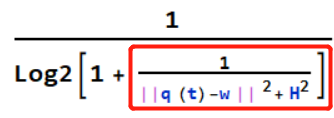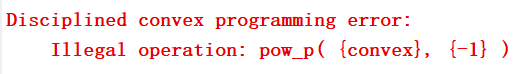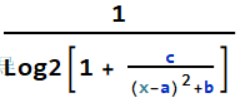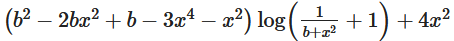# How to express it?q(t) in the expression is the variable, and the whole expression is convex, but when I put it in CVX, it tells me that the content in the red box is “Disciplined convex programming error: Illegal operation: pow_p( {convex}, {-1} )”. How do I represent this whole convex expression？

It is not convex on x>0. Consider a special case 1/log(1+1/(x^2)). When x->0, the second derivative < 0; When x->infinity, the second derivative > 0.

@jackfsuia Edit: My previous calculation. was mistaken. This expression is neither convex nor concave in general.

Thanks a lot for your replying! But the whole expression is indeed a convex one. And my expression is different from the special form that you give. Here is the graph of my expression

Yes! It is indeed convex, but I do not know how to handle the error of the red box in my expression, which is displayed in the following\frac{\partial ^2\frac{1}{\log \left(\frac{C}{x^2}+1\right)}}{\partial x^2}=-\frac{2 C \left(\left(C+3 x^2\right) \log \left(\frac{C}{x^2}+1\right)-4 C\right)}{x^2 \left(C+x^2\right)^2 \log ^3\left(\frac{C}{x^2}+1\right)}. Let’s consider -4 C + (C + 3 x^2) Log(1 + C/x^2). When x->0, the limit is + infinity.When x->infinity, the limit is -C.

2ns derivative of 1/log(1+1/x^2); at x = 0.1 is -1.503. That is a special case of the expression.

This is the second derivative of my expression and it is >0 at any point. The term in the expression is like (x-a)^2+b but not simple x^2, so the second derivative is different.

I mean it is like (x-a)^2+b but not simple x^2, so the second derivative is different.
For this function,when x->any point, second derivative is always > 0
here is the second derivative of my expression.

You need to clarify what is the most general form of expression you need to handle.

if q(t) is a scalar, and H = 0, the expression is not convex, but appears to be convex for H^2 > 0.

If q(t) is a vector of length >= 2 and H = 0, it is neither convex nor concave, but appears to be convex if H > 0.

Edit: Note that I substantively edited the last sentence..

Thank you very much ! q(t) is indeed a a vector of length >= 2.

Let me correct that ( I just edited my preceding post). I think it may be convex for H > 0, even for vector q(t). It is neither convex nor concave when H = 0`.

So even if it is convex, we do not have a way to express it in cvx.

You’ll have to wait to see whether someone comes along who can do it.

OK！Thank you very much for answering so many questions for me！

\frac{\partial ^2\frac{1}{\log \left(\frac{1}{b+x^2}+1\right)}}{\partial x^2}=\frac{2 \left(b^2-2 b x^2+b-3 x^4-x^2\right) \log \left(\frac{1}{b+x^2}+1\right)+8 x^2}{\left(b+x^2\right)^2 \left(b+x^2+1\right)^2 \log ^3\left(\frac{1}{b+x^2}+1\right)}. Can anyone prove \left(b^2-2 b x^2+b-3 x^4-x^2\right) \log \left(\frac{1}{b+x^2}+1\right)+4 x^2>0 for all x or prove the original function is convex? because this is very important for the trajectory design of moving basestation in wireless communication domain, which I believe the poster is working on.

Mr.Stone, could you possibly prove its convexity? Any relavant guidance or idea will be appreciated.

Hi, Jason, could you possibly prove its convexity?

For this,we can prove mathematically that it’s positive or negative. But, it’s a little bit complicated, so I get it by drawing it, and it’s positive when any b>0.